# FJ Matlab Tutorial

## Vectors and Matrices

colon operator :
1:10
ans =
1 2 3 4 5 6 7 8 9 10
v=1:2:10
v =
1 3 5 7 9
Pointwise multiplication and powers of arrays: A.*B and A.^3 (A*B is -matrix- multiplication)
v_sqaured = v.^2
v_sqaured =
1 9 25 49 81
fprintf('norm of v = %f = %f = %f\n', norm(v), sqrt(dot(v,v)), sum(v.^2)^.5)
norm of v = 12.845233 = 12.845233 = 12.845233
linspace()
linspace(1,3,7)
ans =
1.0000 1.3333 1.6667 2.0000 2.3333 2.6667 3.0000
Some functions: size(A), min(A), max(A), sin(A), sum(A), mean(A), isprime(A), A*A prod(A,1), A + 3, A .^2, 2*A, A+A, cross(v,w), find(A==13), union(v,w)
Other ways of creating arrays:
eye(3), zeros(3), ones(3,4), diag([1 2 3]), rand(3,4), randn(1,100), rand(3,4,2) (3-dim array)
m4=magic(4) % magic square!
m4 =
16 2 3 13 5 11 10 8 9 7 6 12 4 14 15 1
sum_cols =sum(m4) % sum of columns
sum_cols =
34 34 34 34
sum_rows = sum(m4, 2) % sum of rows
sum_rows =
34 34 34 34
sum_diagnoal = sum(diag(m4)) % sum of diagonals
sum_diagnoal = 34
A_random = randi([-3,5], 3, 7) % a random 3*7 matrix of integers between -3 and 5
A_random =
4 5 -1 5 5 -2 4 5 2 1 -2 1 0 5 -2 -3 5 5 4 5 2
rank_A = rank(A_random)
rank_A = 3
null(A): An orthornomal basis for the null space of matrix A
null_A = null(A_random)
null_A =
0.2978 -0.1155 0.1112 -0.6674 -0.6603 -0.3485 0.2502 0.1597 -0.4282 -0.3650 -0.5261 -0.2239 0.3715 -0.4159 -0.0028 -0.0410 -0.2799 0.7438 -0.0437 -0.1762 -0.0991 -0.0745 0.7979 -0.0322 0.2565 0.0128 -0.0984 0.6672
matrix_dimensions = size(null_A)
matrix_dimensions =
7 4
% verify orthonormality by showing null_A' * null_A = 4*4 identity matrix
norm_of_difference = norm(null_A' * null_A - eye(4))
norm_of_difference = 2.3957e-16
fprintf('verifying orthonormality, i.e., null_A'' * null_A = eye(4). In fact norm of the difference = %.15f\n', norm_of_difference)
verifying orthonormality, i.e., null_A' * null_A = eye(4). In fact norm of the difference = 0.000000000000000

## Indexing: accessing array elements

p5 = pascal(5) % binomial coefficient
p5 =
1 1 1 1 1 1 2 3 4 5 1 3 6 10 15 1 4 10 20 35 1 5 15 35 70
p5([1 3 5], [1 3]) % submatrix
ans =
1 1 1 6 1 15
p5(3:5,:) % submatrix
ans =
1 3 6 10 15 1 4 10 20 35 1 5 15 35 70
Cholesky decomposition of a symmetric matrix: chol(A)
c = chol(p5)
c =
1 1 1 1 1 0 1 2 3 4 0 0 1 3 6 0 0 0 1 4 0 0 0 0 1
is_correct = isequal(c'*c, p5) %verify correctness of Cholesky decomposition
is_correct =
1
logical indexing
p5(p5 < 5)=0
p5 =
0 0 0 0 0 0 0 0 0 5 0 0 6 10 15 0 0 10 20 35 0 5 15 35 70
indexing to new shape and end keyword
a = 1:10
a =
1 2 3 4 5 6 7 8 9 10
a([3 4 6 8; 4 7 8 9]) % preserving shape of indexing
ans =
3 4 6 8 4 7 8 9
a(3:end) %end key word
ans =
3 4 5 6 7 8 9 10
a(1:4:end)
ans =
1 5 9

## Reshaping Arrays

Concatenating matrices
A = ones(2,3)*5; % 2 by 3 matrix of all 5's.
B=rand(2,3); % 2 by 3 matrix of uniform [0,1] random numbers
C=[A;B] % cat horizontal (B below A)
C =
5.0000 5.0000 5.0000 5.0000 5.0000 5.0000 0.0357 0.9340 0.7577 0.8491 0.6787 0.7431
D=[A,B] % cat vertical (B to right of A)
D =
5.0000 5.0000 5.0000 0.0357 0.9340 0.7577 5.0000 5.0000 5.0000 0.8491 0.6787 0.7431
Deleting rows and columns
D(:, 4) = [] % deleting one column
D =
5.0000 5.0000 5.0000 0.9340 0.7577 5.0000 5.0000 5.0000 0.6787 0.7431
C([2 3], :) = [] % deleting two rows
C =
5.0000 5.0000 5.0000 0.8491 0.6787 0.7431
transpose, revel, reshape
C_transpose = C' % transpose
C_transpose =
5.0000 0.8491 5.0000 0.6787 5.0000 0.7431
C_ravel = C(:)' % raveling
C_ravel =
5.0000 0.8491 5.0000 0.6787 5.0000 0.7431
C(3,6)=99 % assigning out of array bounds expand array
C =
5.0000 5.0000 5.0000 0 0 0 0.8491 0.6787 0.7431 0 0 0 0 0 0 0 0 99.0000
m4_resphape = reshape(magic(4), [2,8]) % reshaping a 4*4 matrix into a 2*8 matrix
m4_resphape =
16 9 2 7 3 6 13 12 5 4 11 14 10 15 8 1

## Plotting

ezplot() - just give (optionally) the range (usually as [a,b]). Has symbolic version too: syms x y; ezplot(x^2)
ezplot('gamma(x)',[-4,5]), grid on % gamma function: has poles at 0 and negative integers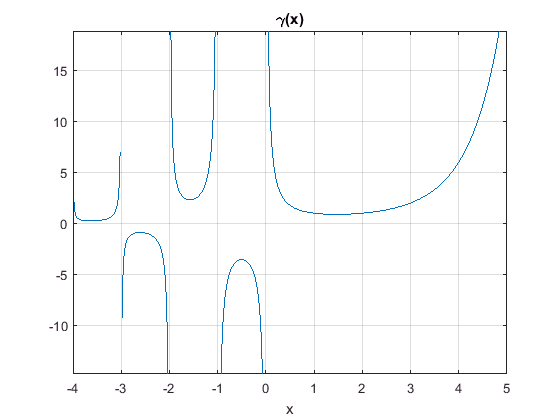ezplot('(x^2+y^2)^2=20*(x^2-y^2)') % implicit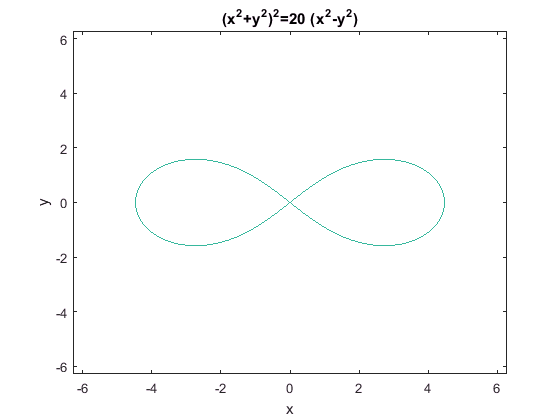parametric 3D: ezplot3() (ezplot() does 2D parametric), e.g., ezplot('cos(3*t)', 'sin(2*t)')
ezplot3('sin(t)','cos(t)','t',[0,6*pi])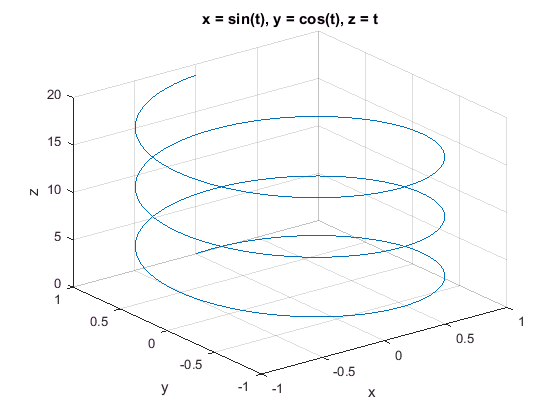x-y plotting: plot() (also fplot())
x = -3:.1:3;
y = exp(-x.^2);
plot(x,y)
grid on, xlabel('x'), ylabel('y'), title('exp(-x^2) Graph')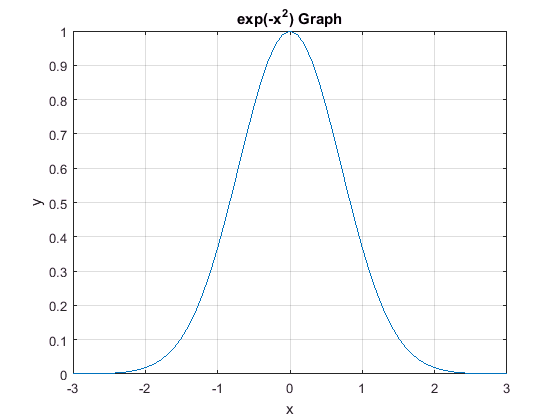Plotting two curves Either use "hold on" or plot(x,y1,x,y2)
x = -5:.2:5;
plot(x, sin(x), x, cos(x), '--'), legend('Sin(x)', 'Cos(x)'), grid on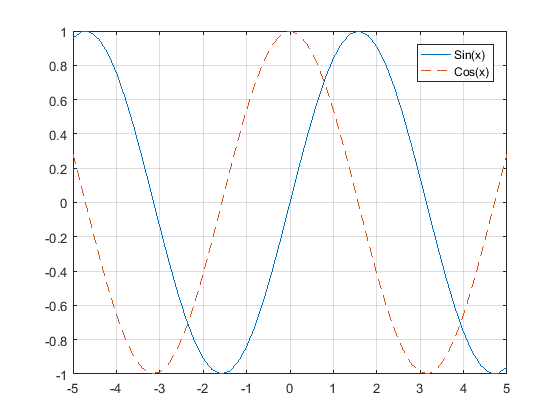Surface plot: ezsurf() or ezsurfc() to also see the contours
ezsurf('sin(sqrt(x^2+y^2)) / sqrt(x^2+y^2)') % may use a different domain , e.g. [-7,7] (applicable to both x y), or [-7,7,-4,4]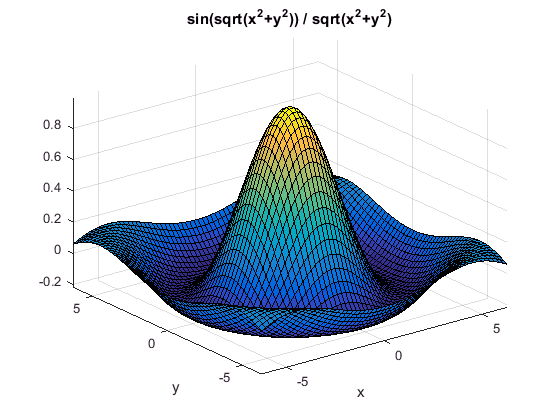Surface plot: surf() or surfc() to also see the contours
[x,y] = meshgrid(-2:.1:2);
g = x .* exp(-x.^2 - y.^2);
surfc(x, y, g)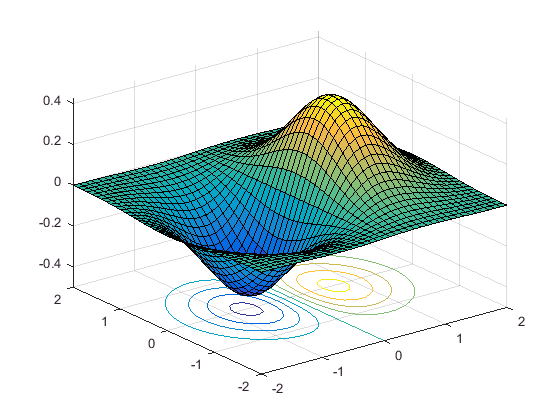ezsurf('s*sin(t)','s*cos(t)', 't', [-1,1,0,8])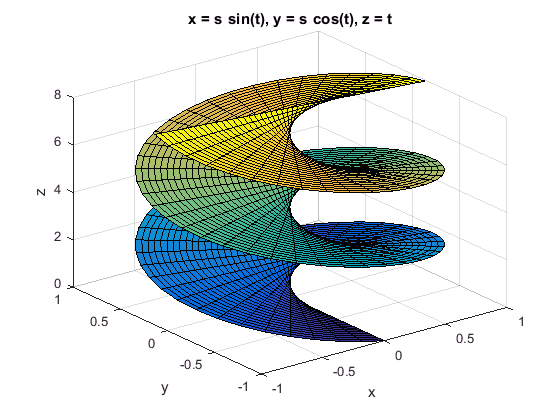Contour plot: ezcontourf()
ezcontourf('sin(3*x)*cos(x+y)',[0,5])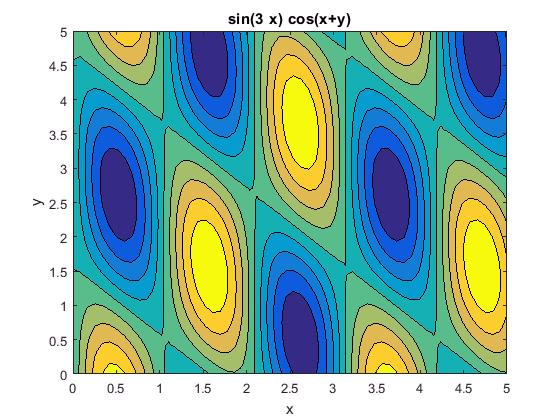% colormap('spring') % changing color
Polar coordinates: polar() or simply ezpolar('sin(4*t)')
ezpolar('sin(4*t)')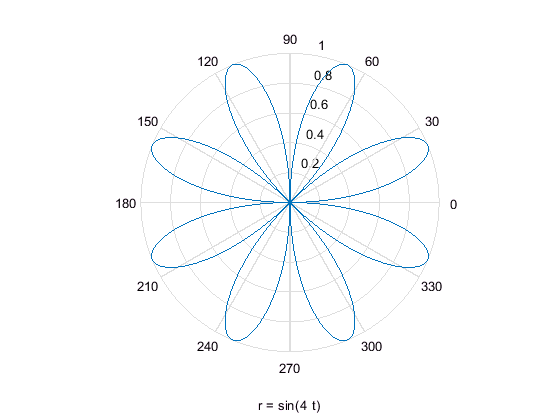%t = 0:0.01:2*pi;
%r = sin(4*t);
%polar(t, r, 'red') % this renders in red
Histogram: histogram()
x = randn(10000,1); %10000 random normals
histogram(x)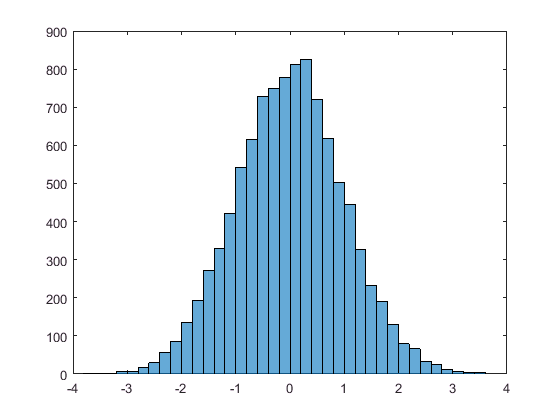Scatter Plot : scatter()
x = linspace(0,10, 50);
y = 4 + x/2 + 2*randn(1,50);
scatter(x,y), lsline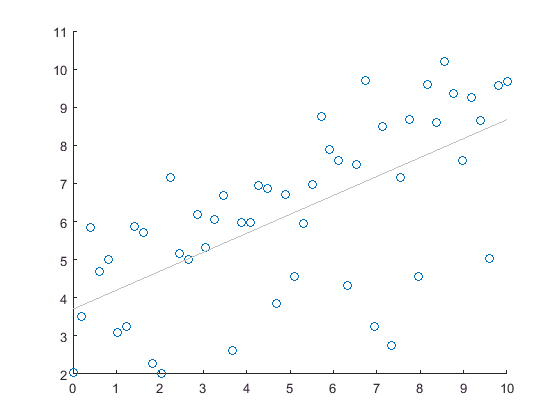## Random numbers

First, initialize the random number generator to make the results in this example repeatable.
rng(0,'twister') % reset the seed
r_uniform = rand(1,6) % uniform [0,1]
r_uniform =
0.8147 0.9058 0.1270 0.9134 0.6324 0.0975
r_integers = randi(12,2,9) % 2 by 9 matrix of random integer between 1 and 12
r_integers =
4 12 2 12 10 6 10 8 11 7 12 12 6 2 11 12 1 12
r_normal = randn(1,6) % normal
r_normal =
0.4889 1.0347 0.7269 -0.3034 0.2939 -0.7873
mean_std = [mean(r_normal), std(r_normal)] % mean and std
mean_std =
0.2423 0.6759
r_permutation = randperm(15,10) % a length 10 random permutation of 15 element
r_permutation =
9 6 10 12 3 13 4 15 11 2

## Linear Equations

matrix multiplication, det(), etc.
pointwise_add = [2 3 -2] + [4 5 7] %pointwise multiplication (for vectors, matrices (all arrays))
6 8 5
pointwise_mult = [2 3 -2] .* [4 5 7] %pointwise multiplication (for vectors, matrices (all arrays))
pointwise_mult =
8 15 -14
A = magic(3);
matrix_multp = A * A % = A^2, matrix multiplication
matrix_multp =
91 67 67 67 91 67 67 67 91
det_A = det(A) % similarly trace(A)
det_A = -360
inverse and solution
inverse = inv(A) % inverse
inverse =
0.1472 -0.1444 0.0639 -0.0611 0.0222 0.1056 -0.0194 0.1889 -0.1028
x = linsolve(A,[1 0 0]') %linear solve
x =
0.1472 -0.0611 -0.0194
x= A \ [1, 0, 0]' % same linear solve, but shorter
x =
0.1472 -0.0611 -0.0194
eigenvalues and eigenvectors
eigenvalues = eig(A) % eigenvalues
eigenvalues =
15.0000 4.8990 -4.8990
[V,D] = eig(A) % eigenvectors and eigenvalues
V =
-0.5774 -0.8131 -0.3416 -0.5774 0.4714 -0.4714 -0.5774 0.3416 0.8131
D =
15.0000 0 0 0 4.8990 0 0 0 -4.8990

## Least Square Linear Regression:

short way using X \y
x1 = [.2 .5 .6 .8 1.0 1.1]';
x2 = [.1 .3 .4 .9 1.1 1.4]';
X = [ones(size(x1)) x1 x2];
y = [.17 .26 .28 .23 .27 .34]';
b = X \ y
b =
0.1203 0.3284 -0.1312
long way using lscov(X,y). But can also return standard error of coefficients and mean square error
[b,se_b,mse] = lscov(X,y)
b =
0.1203 0.3284 -0.1312
se_b =
0.0643 0.2267 0.1488
mse = 0.0015
polynomial curve fitting
% cubic polynomial fit
x=.07*(1:30);
y=(x-.2).*(x-1).*(x-2)+.3*randn(1,30); % randoms added to a cubic
poly_coefs = polyfit(x,y,3) % polynomial coefficients
poly_coefs =
1.1571 -3.7964 3.2043 -0.5303
y_fitted = polyval(poly_coefs,x);
plot(x,y,'o', x, y_fitted, '*-', 'Markersize', 8, 'MarkerFaceColor', 'b')
title('Cubic polynomial least squared fit to data'), grid on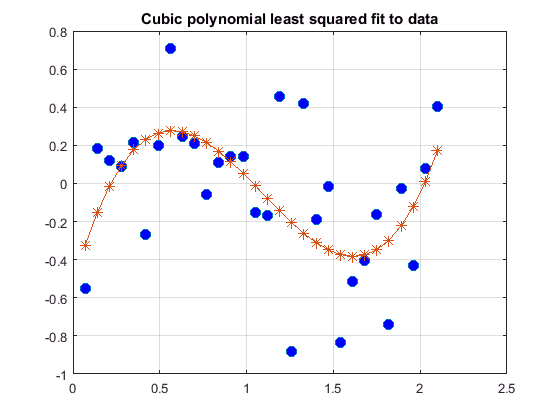## Interpolation

Cubic spline interpolation
x = [0 1 2.5 3.6 5 7 8.3 10];
y = sin(x);
xx = 0:.25:10;
yy = spline(x, y, xx);
plot (x, y, 'o', xx, yy, xx, sin(xx), '--b', 'Linewidth', 1.5, 'Markersize', 8)
legend('sin data', 'cubic spline', 'sin fit' , 'Location', 'southeast')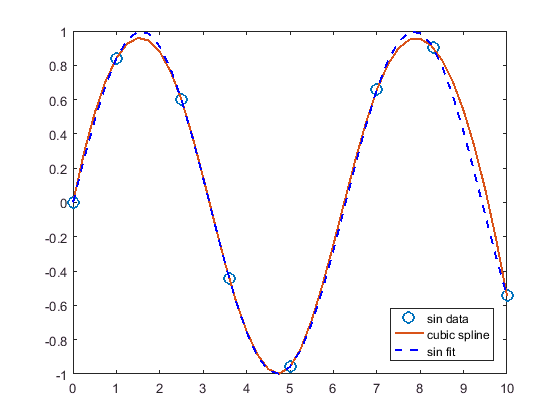## Optimization

For maximizing f(x), minimize -f(x)
one variable: fminbnd(). Can further use
opts = optimset('Display','iter');
To find the minimum of the humps function in the range (0.3,1), use pointer to function @humps
x = fminbnd(@humps,0.3,1) % @humps is pointer to the humps(x) funtion
x = 0.6370
several variables: fminsearch() Find a minimum for "three_var()" function using x = -0.6, y = -1.2, z = 0.135 as starting values.
v = [-0.6 -1.2 0.135]; %initial search
fun = @(v) v(1).^2 + 2.5*sin(v(2)) - v(3)^2*v(1)^2*v(2)^2;
fminsearch(fun, v)
ans =
0.0000 -1.5708 0.1803
%fminsearch(@three_var, v) % here three_var() is defined in an m file
Linear programming
min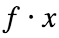s.t.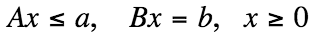f = [-2 -1 -4 0 -2];
A = [4 2 3 1 0; 3 2 1 -3 4];
a = [12 8];
B = [0 1 -1 0 0; 0 0 0 1 -2];
b = [1 0];
lb = [0 0 0 0 0];
[x fval] =linprog(f, A, a, B, b, lb)
Optimization terminated.
x =
0.0000 1.8211 0.8211 5.8946 2.9473
fval = -11.0000

## Nonlinear Least Square

lsqcurvefit(): from Optimization toolbox. Below solving a Physical BioChemistry problem
xdata = [50,55,60,65,70,75,80,85,90];
ydata = [3.4,3.3,3.2,2.5,1.4,.25,.12,-0.1,-0.25];
logistic_fun = @(x,xdata)(x(1)-x(2))./(1+exp(x(3)*(xdata-x(4)))) + x(2);
x0 = [3.5,-0.3,0.25, 69.2];
options = optimoptions('lsqcurvefit','Display','none'); % so as to suppress warning output
x = lsqcurvefit(logistic_fun,x0,xdata,ydata, [], [], options) % x = lsqcurvefit(fun,x0,xdata,ydata)
x =
3.4006 -0.1609 0.2908 68.9322
times = linspace(xdata(1),xdata(end));
plot(xdata,ydata,'ko',times,logistic_fun(x,times),'b-')
grid on, title('Nolinear least square fit to the Logistic Curve')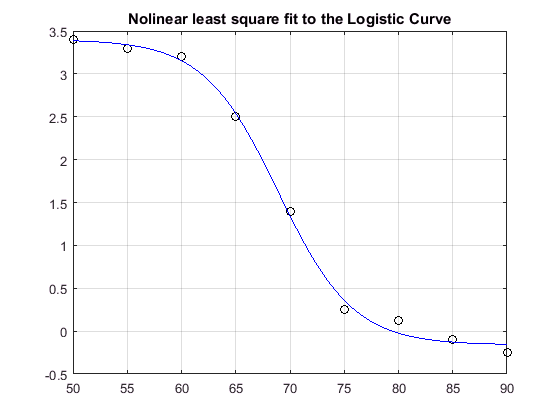## Numeric Root Finding

Numeric one equation in one unknown: fzero()
fzero() admits an interval or point as initial search, and has options
Calculate π by finding the zero of the sine function near 3.
fun = @sin; % function
x0 = 3; % initial point
x = fzero(fun,x0)
x = 3.1416
Find the zero of cosine between 1 and 2.
fun = @cos; % function
x0 = [1 2]; % initial interval
[x,fval,exitflag,output] = fzero(fun,x0)
x = 1.5708
fval = 6.1232e-17
exitflag = 1
output =
intervaliterations: 0 iterations: 5 funcCount: 7 algorithm: 'bisection, interpolation' message: 'Zero found in the interval [1, 2]'
root of polynomials root()
roots([1 0 -2 -5]) % x^3 -2x -5
ans =
2.0946 + 0.0000i -1.0473 + 1.1359i -1.0473 - 1.1359i
root of function with parameter
myfun = @(x,c) cos(c*x); % parameterized function
c = 2; % parameter
fun = @(x) myfun(x,c); % function of x alone
x = fzero(fun, 0.1)
x = 0.7854
numeric multivariable: fsolve() of Optimization Toolbox. Pass initial guess
root2d=@(x)[ exp(-exp(-x(1)+x(2))) - x(2)*(1+x(1)^2), x(1)*cos(x(2)) + x(2)*sin(x(1)) - 0.5];
x = fsolve(root2d, [0,0])
Equation solved. fsolve completed because the vector of function values is near zero as measured by the default value of the function tolerance, and the problem appears regular as measured by the gradient. <stopping criteria details>
x =
0.3931 0.3366
To supress warning output, call fsolve() with option:
options = optimoptions('fsolve','Display','none');
x = fsolve(root2d, [0,0], options)
x =
0.3931 0.3366

## Symbolic Root Finding

Symbolic one equation in one unknown: solve()
syms a b c x
sol = solve(a*x^2 + b*x + c == 0) % implicitly x is assumed as unknown
sol =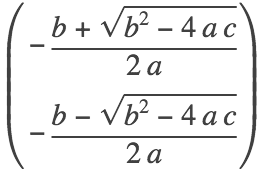sola = solve(a*x^2 + b*x + c == 0, a)
sola =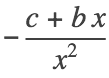Symbolic numeric one equation in one unknown: vpasolve()
syms x
vpasolve(200*sin(x) == x^3 - 1, x)
ans =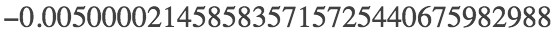vpasolve(200*sin(x) == x^3 - 1, x, 4) % with initial value 4
ans =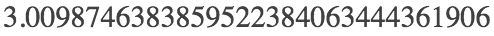vpasolve(200*sin(x) == x^3 - 1, x, [-5,-3]) % in interval [-4,-3]
ans =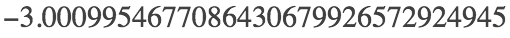Symbolic two equations in two unknowns: solve()
syms u v
[u,v]=solve([2*u^2 + v^2 == 1, u - 2*v == 0], [u, v]) % 2 dimensional
u =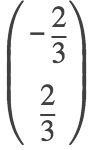v =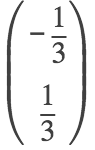Symbolic numeric two equations in two unknowns: vpasolve()
syms x y
[sol_x, sol_y] = vpasolve([x*sin(10*x) == y^3, y^2 == exp(-2*x/3)], [x, y])
sol_x =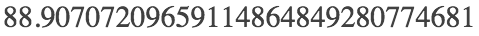sol_y =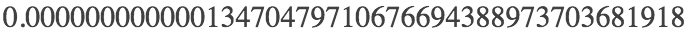## Symbolic Calculus

limit
syms x
a = limit((1-cos(x))/x^2, 0) %limit
a =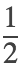b = limit(abs(x)/x, x, 0, 'right')
b =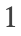series
syms k n x
S1 = symsum(k^2, k, 0, n) % finite sum
S1 =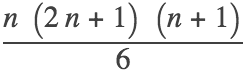S2 = symsum(1/k^2, k, 1, Inf) % infinite series
S2 =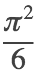S3 = symsum(x^k,k,0,Inf) % geometric series
S3 =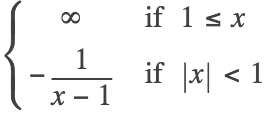derivative
syms t
f = 2*t^(-2) +t^3* log(t);
diff(f) % derivative
ans =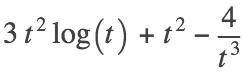diff(f,2) % second derivative
ans =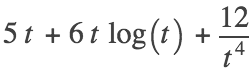integral
Calculate. Verfiy equalssyms x a
syms n integer;
I = int(x*(a-x)*sin(n*pi*x/a),[0,a]);
definite_integral = simplify(I)
definite_integral =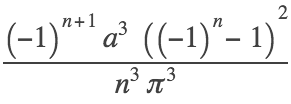syms t a
indefinite_integral = int(1/(a+cos(t))) % substitute u=tan(x/2)
indefinite_integral =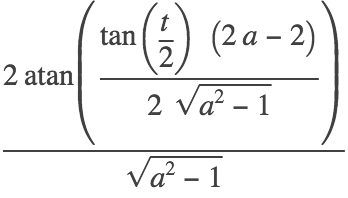numerical integration
Compute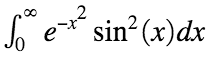fun = @(x) exp(-x.^2).*sin(x).^2;
integral(fun,0,Inf)
ans = 0.2801
algebra
syms x y
expand(cos(x+y))
ans =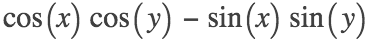factor(x^3 - y^3)
ans =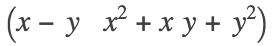simplify((x^4-16)/(x^2-4))
ans =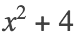## Symbolic Vector Calculus

curl(F) of a vector field F.
syms x y z
F = [x^3*y^2*z, y^3*z^2*x, z^3*x^2*y];
curl_F = curl(F, [x, y, z])
curl_F =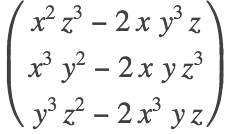potential function for a conservative vector field F : given F with curl(F)=0 find function f with grad(f) = F
syms x y z F f
F = [x; y; z*exp(z)]
F =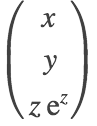curl_F = curl(F)' % verify cur(F) = 0
curl_F =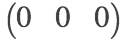f = potential(F, [x y z])
f =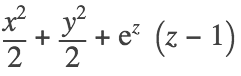grad_f_minus_F =potential vector field for an incompressible vector field F: given F with div(F)=0, find P with curl(P) = F
syms x y z F P
F = [2*y^3 - 4*x*y; 2*y^2 - 16*z^2+18; -32*x^2 - 16*x*y^2];
div_F = divergence(F) % verfiry div(F)=0
div_F =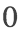P = vectorPotential(F, [x y z]) % solve for the potential P
P =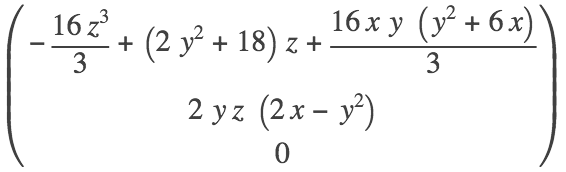curl_P_minus_F = simplify(curl(P)-F)' % verify curl(P) = F
curl_P_minus_F =syms x y z
f(x,y,z)=1/sqrt(x^2+y^2+z^2)
f(x, y, z) =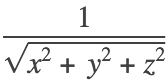L = laplacian(f, [x y z]); % In the sense of generalized functions, L is the Dirac delta function at the origin
L_f = simplify(L)
L_f(x, y, z) =## Symblic Ordinary Differential Equations

First order ODE: nonlinear, variable-coefficient, homogenous
s = dsolve('Dy = (sin(t))^2*exp(y)')
s =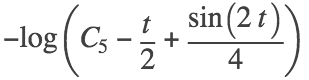s= dsolve('Dy = (sin(t))^2*exp(y)', 'y(0)=1')
s =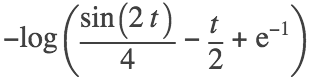First order ODE: nonlinear (nonlinear in y' - solution not unique)
s=dsolve('(Dy+y)^2 == 1')
s =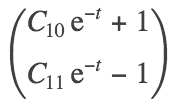% alternative syntax
syms y(t)
y(t) = dsolve((diff(y,t) + y)^2 == 1, y(0) == 2)
y(t) =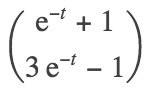Second order ODE: linear, inhomogeneous
s=dsolve('D2y = cos(2*t) - y', 'y(0) = 1', 'Dy(0) = 0');
s= simplify(s)
s =